# Tilt Angle & Distance Meter Using Accelerometer & Ultrasonic Sensor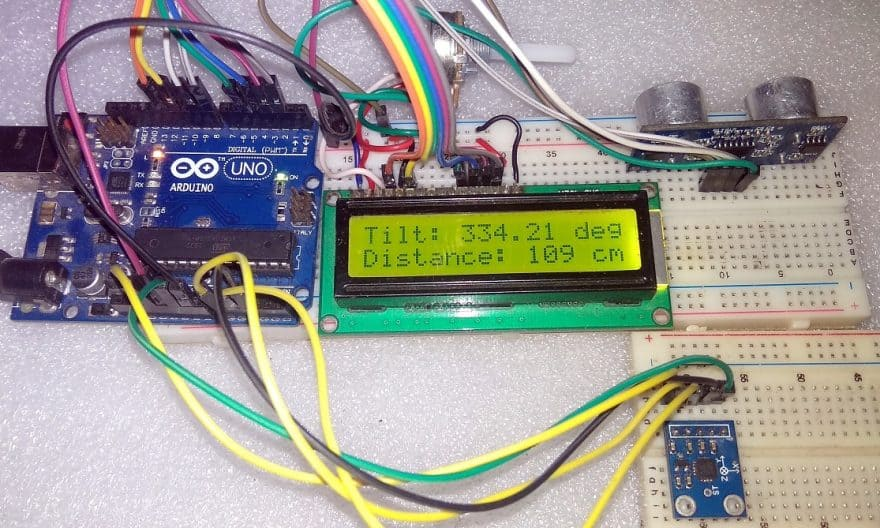(Last Updated On: March 17, 2019)

### Acceleration Measurement with Accelerometer ADXL335 & Arduino:

In this post we will learn about Tilt Angle & Distance Meter Using Accelerometer & Ultrasonic Sensor with Arduino. We have used ADXL335 3 axis accelerometer for measuring the angle in 360 degree dimension. Similarly we have used HC-SR04 Ultrasonic Sensor to measure distance up to 400 cm. The measured angle and distance is displayed on 16*2 LCD Display. You can even use ADXL345 Digital Accelerometer with I2C interface.

Here we will be interfacing ADXL335 Accelerometer and Ultrasonic Sensor HC-SR04 with Arduino to measure the angle and distance. But before getting started you can visit our earlier post to learn more about accelerometer and Ultrasonic Sensor.

### Components Required:

1. Arduino UNO Board (Buy Online from Amazon)
2. ADXL335 3 Axis Accelerometer (Buy Online from Amazon)
3. HC-SR04 Ultrasonic Sensor (Buy Online from Amazon)
4. 16×2 LCD Display (Buy Online from Amazon)
5. Breadboard
6. Jumper Wires
7. 5 Volt Power Supply

### What is an Accelerometer?

Accelerometers are devices that measure acceleration, which is the rate of change of the velocity of an object. They measure in meters per second squared (m/s2) or in G-forces (g). A single G-force for us here on planet Earth is equivalent to 9.8 m/s2, but this does vary slightly with elevation (and will be a different value on different planets due to variations in gravitational pull). Accelerometers are useful for sensing vibrations in systems or for orientation applications.

### ADXL335 3 Axis Accelerometer:

#### Introduction: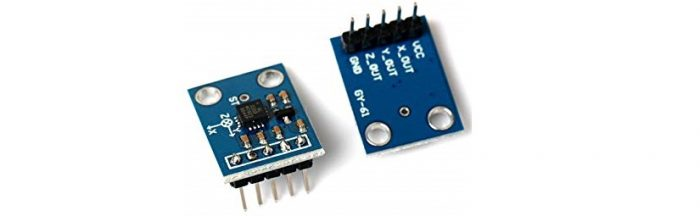This Accelerometer module is based on the popular ADXL335 three-axis analog accelerometer IC, which reads off the X, Y and Z acceleration as analog voltages. By measuring the amount of acceleration due to gravity, an accelerometer can figure out the angle it is tilted at with respect to the earth. By sensing the amount of dynamic acceleration, the accelerometer can find out how fast and in what direction the device is moving. Using these two properties, you can make all sorts of cool projects, from musical instruments (imagine playing and having the tilt connected to the distortion level or the pitch-bend) to a velocity monitor on your car (or your children’s car). The accelerometer is very easy interface to an Arduino Micro-controller using 3 analog input pins, and can be used with most other micro controllers, such as the PIC or AVR.

#### Working of ADXL335 Accelerometer:

The most commonly used device is the piezoelectric accelerometer. As the name suggests, it uses the principle of piezoelectric effect. The device consists of a piezoelectric quartz crystal on which an accelerative force, whose value is to be measured, is applied.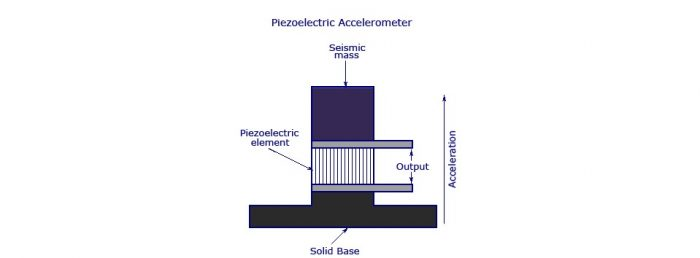Due to the special self-generating property, the crystal produces a voltage that is proportional to the accelerative force. The working and the basic arrangement is shown in the figure below.

### HC-SR04 Ultrasonic Sensor:

#### What is Ultrasonic Sensor?

The HC-SR04 ultrasonic sensor uses sonar to determine distance to an object like bats do. It offers excellent non-contact range detection with high accuracy and stable readings in an easy-to-use package.From 2cm to 400 cm or 1” to 13 feet. Its operation is not affected by sunlight or black material like sharp rangefinders are (although acoustically soft materials like cloth can be difficult to detect). It comes complete with ultrasonic transmitter and receiver module.

#### How does HC-SR04 Ultrasonic Sensor Works?

Ultrasonic sensors emit short, high-frequency sound pulses at regular intervals. These propagate in the air at the velocity of sound. If they strike an object, then they are reflected back as echo signals to the sensor, which itself computes the distance to the target based on the time-span between emitting the signal and receiving the echo.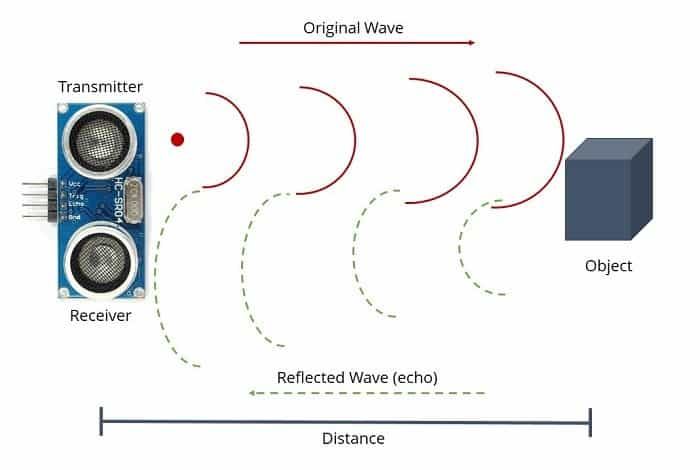We will have to convert this time into cm to calculate the distance traveled. We will use the following equation to calculate the distance.

`S = v * t`

Ultrasonic wave is basically a sound wave which travels at a speed of 340 m/s (0.034 cm/s). The ultrasonic sensor is measuring the time it takes to hit the object and then come back but we need only time that it takes to hit the object. So, we will divide it by 2.

`S = (t * 0.034)/2`

### Circuit Diagram & Connection for Acceleration Measurement:

Here is a circuit diagram for Tilt Angle & Distance Meter Using Accelerometer & Ultrasonic Sensor. Assemble the circuit as shown in the figure below.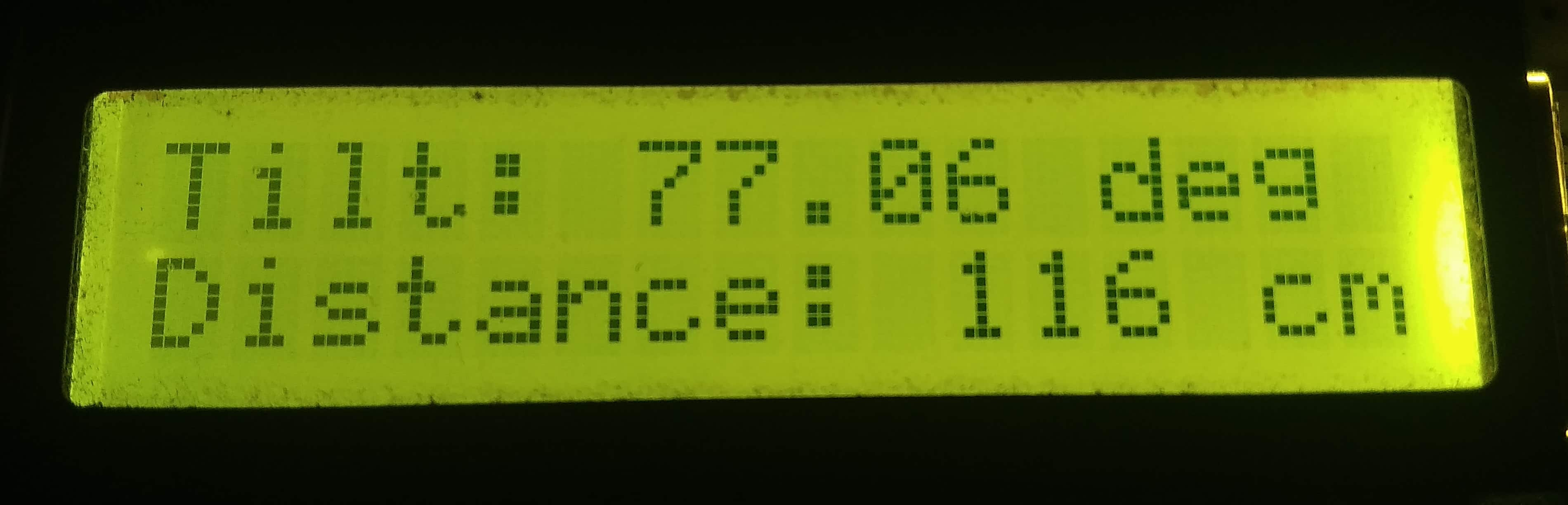### Source Code/ Programs:

```#include <LiquidCrystal.h>
#define ADC_ref 5 // ADC reference Voltage
#define zero_x 1.799
#define zero_y 1.799
#define zero_z 1.799
#define echoPin 8
#define trigPin 9
#define selectSwitch 1
#define sensitivity_x 0.4
#define sensitivity_y 0.4
#define sensitivity_z 0.4
unsigned int value_x;
unsigned int value_y;
unsigned int value_z;
float xv;
float yv;
float zv;
float angle;
LiquidCrystal lcd(12,11,5,4,3,2);
void setup()
{
analogReference(ADC_ref);
pinMode(selectSwitch,INPUT);
pinMode(trigPin,OUTPUT);
pinMode(echoPin,INPUT);
lcd.clear();
lcd.begin(16,2);
lcd.print("Tilt & Distance");
lcd.setCursor(0,2);
lcd.print(" Measurement ");
delay(3000);
lcd.clear();
}
void loop()
{
int distance,duration;
value_x = analogRead(A0);
value_y = analogRead(A1);
value_z = analogRead(A2);
xv=(value_x/1024.0*ADC_ref-zero_x)/sensitivity_x;
yv=(value_y/1024.0*ADC_ref-zero_y)/sensitivity_y;
zv=(value_z/1024.0*ADC_ref-zero_z)/sensitivity_z;
angle =atan2(-yv,-zv)*57.2957795+180;
if(digitalRead(selectSwitch)==HIGH)
{
lcd.setCursor(0,0);
lcd.print("Tilt: ");
lcd.print(angle);
lcd.print(" deg");
digitalWrite(trigPin, HIGH);
delayMicroseconds(1000);
digitalWrite(trigPin, LOW);
duration = pulseIn(echoPin, HIGH);
distance = (duration/2) / 29.1;
if (distance >= 400 || distance <= 0)
{
lcd.setCursor(0,1);
lcd.print("Out of Range");
}
else
{
lcd.setCursor(0,1);
lcd.print("Distance: ");
lcd.print(distance);
lcd.print(" cm ");
}
delay(1000);
lcd.clear();
}
else
{
lcd.setCursor(0,0);
lcd.print("X=");
lcd.print(xv);
lcd.print(" Y=");
lcd.print(yv);
lcd.setCursor(0,1);
lcd.print(" Z= ");
lcd.print(zv);
delay(1000);
}
}```

### Recommended For You